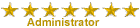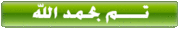solid - كتاب Finite Element Method Volume 2 - Solid Mechanics
 منتدى هندسة الإنتاج والتصميم الميكانيكى بسم الله الرحمن الرحيم أهلا وسهلاً بك زائرنا الكريم نتمنى أن تقضوا معنا أفضل الأوقات وتسعدونا بالأراء والمساهمات إذا كنت أحد أعضائنا يرجى تسجيل الدخول أو وإذا كانت هذة زيارتك الأولى للمنتدى فنتشرف بإنضمامك لأسرتنا وهذا شرح لطريقة التسجيل فى المنتدى بالفيديو : http://www.eng2010.yoo7.com/t5785-topic وشرح لطريقة التنزيل من المنتدى بالفيديو: http://www.eng2010.yoo7.com/t2065-topic إذا واجهتك مشاكل فى التسجيل أو تفعيل حسابك وإذا نسيت بيانات الدخول للمنتدى يرجى مراسلتنا على البريد الإلكترونى التالى : Deabs2010@yahoo.comمنتدى هندسة الإنتاج والتصميم الميكانيكى :: المنتديات الهندسية :: منتدى الكتب والمحاضرات الهندسية :: منتدى الكتب والمحاضرات الهندسية الأجنبية Tweetشاطر

# كتاب Finite Element Method Volume 2 - Solid Mechanicsكاتب الموضوعرسالة
مدير المنتدىعدد المساهمات : 15959
التقييم : 27167
تاريخ التسجيل : 01/07/2009
العمر : 31
الدولة : مصر
العمل : مدير منتدى هندسة الإنتاج والتصميم الميكانيكى
الجامعة : المنوفيةموضوع: كتاب Finite Element Method Volume 2 - Solid Mechanicsالجمعة 20 ديسمبر 2013, 2:44 pm أخوانى فى اللهأحضرت لكم كتابFinite Element Method Volume 2 - Solid Mechanicsويتناول الموضوعات الأتية :1.  General problems in solid mechanics and non-linearity 1.1  Introduction 1.3  Non-linear quasi-harmonic field problems 1.5  Concluding remarks Small deformation non-linear solid mechanics problems Some typical examples of transient non-linear calculations References 2.  Solution of non-linear algebraic equations 2.1  Introduction 2.2  Iterative techniques References 3.  Inelastic and non-linear materials Introduction Viscoelasticity - history dependence of deformation Classical time-independent plasticity theory Computation of stress increments Isotropic plasticity models Generalized plasticity - non-associative case Some examples of plastic computation Basic formulation of creep problems Viscoplasticity - a generalization Some special problems of brittle materials Non-uniqueness and localization in elasto-plastic deformations Adaptive refinement and localization (slip-line) capture Non-linear quasi-harmonic field problems References 4.  Plate bending approximation: thin (Kirchhoff) plates and C, continuity requirements viii  Contents Introduction The plate problem: thick and thin formulations Rectangular element with corner nodes (12 degrees of freedom) Quadrilateral and parallelogram elements Triangular element with corner nodes (9 degrees of freedom) Triangular element of the simplest form (6 degrees of freedom) The patch test - an analytical requirement Numerical examples General remarks Singular shape functions for the simple triangular element An 18 degree-of-freedom triangular element with conforming shape functions Compatible quadrilateral elements Quasi-conforming elements Hermitian rectangle shape function The 21 and 18 degree-of-freedom triangle Mixed formulations - general remarks Hybrid plate elements Discrete Kirchhoff constraints Rotation-free elements Inelastic material behaviour Concluding remarks - which elements? References 5.  ‘Thick’ Reissner-Mindlin  plates - irreducible and mixed formulations 5.1  Introduction 5.10  Forms without rotation parameters 5.1 1  Inelastic material behaviour The irreducible formulation - reduced integration Mixed formulation for thick plates The patch test for plate bending elements Elements with discrete collocation constraints Elements with rotational bubble or enhanced modes Linked interpolation - an improvement of accuracy Discrete ‘exact’ thin plate limit Performance of various ‘thick‘ plate elements - limitations of thin plate theory Concluding remarks - adaptive refinement References 6.  Shells as an assembly of flat elements 6.1  Introduction 6.4  Local direction cosines Stiffness of a plane element in local coordinates Transformation to global coordinates and assembly of elements ‘Drilling’ rotational stiffness - 6 degree-of-freedom  assembly Elements with mid-side slope connections only  Contents  ix 6.7  Choice of element 6.8  Practical examples References 7.  Axisymmetric shells  244 7.1  Introduction  244 7.2  Straight element  245 7.3  Curved elements  25 1 7.4  Independent slope-displacement interpolation with penalty functions (thick or thin shell formulations)  26 1 References  264 8.  Shells as a special case of three-dimensional analysis - Reissner-Mindlin assumptions 8.1  Introduction 8.5  Convergence 8.6  Inelastic behaviour 8.7  Some shell examples 8.8  Concluding remarks Shell element with displacement and rotation parameters Special case of axisymmetric, curved, thick  shells Special case of thick plates References 9.  Semi-analytical finite element processes - use of orthogonal functions and ‘finite strip’ methods 9.1  Introduction 9.2  Prismatic bar 9.3  Thin membrane box structures 9.8  Concluding remarks Plates and boxes with flexure Axisymmetric solids with non-symmetrical load Axisymmetric shells with non-symmetrical load Finite strip method - incomplete decoupling References 10. Geometrically non-linear problems - finite deformation 10.1  Introduction 10.2  Governing equations 10.8  Contact problems 10.9  Numerical examples Variational description for finite deformation A three-field mixed finite deformation formulation A mixed-enhanced finite deformation formulation Forces dependent on deformation - pressure loads Material constitution for finite deformation x  Contents 10.10 Concluding remarks References 1 1. Non-linear structural problems - large displacement and instability 1 1.1  Introduction 11.7  Shells 11.8  Concluding remarks Large displacement theory of beams Elastic stability - energy interpretation Large displacement theory of thick plates Large displacement theory of thin plates Solution of large deflection problems References 12. Pseudo-rigid and rigid-flexible bodies 12.1  Introduction 12.2  Pseudo-rigid motions 12.3  Rigid motions  12.5  Multibody coupling by joints 12.6  Numerical  examples Connecting a rigid body to a flexible body References 13. Computer procedures for finite element analysis 13.1  Introduction 13.2 13.3  Solution of non-linear problems 13.4  Restart option 13.5  Solution of example problems 13.6  Concluding remarks Description of additional program features References Appendix A:  Invariants of second-order tensors A. 1  Principal invariants A.2  Moment invariants A.3  Derivatives of invariants Author index Subject index  Volume 1: The basis 1. Some preliminaries: the standard discrete system 2. A direct approach to problems in elasticity 3. Generalization of the finite element concepts.  Galerkin-weighted residual and 4. Plane stress and plane strain 5. Axisymmetric stress analysis 6. Three-dimensional stress analysis 7. Steady-state field problems - heat conduction, electric and magnetic potential, 8. ‘Standard’ and ‘hierarchical’ element shape functions: some general  families of 9. Mapped elements and numerical integration - ‘infinite’ and ‘singularity’ elements variational approaches fluid flow, etc C, continuity 10. The patch test, reduced integration, and non-conforming elements 1 1. Mixed formulation and constraints - complete field methods 12. Incompressible problems, mixed methods and other procedures of solution 13. Mixed formulation and constraints - incomplete (hybrid) field methods, bound- 14. Errors, recovery processes and error estimates 15. Adaptive finite element refinement 16. Point-based approximations; element-free Galerkin - and other meshless methods 17. The time dimension - semi-discretization  of field and dynamic  problems and 18. The time dimension - discrete approximation in time 19. Coupled systems 20. Computer procedures for finite element analysis Appendix A. Matrix algebra Appendix B. Tensor-indicia1 notation in the approximation of elasticity problems Appendix C. Basic equations of displacement  analysis Appendix D. Some integration formulae for a triangle Appendix E.  Some integration formulae for a tetrahedron Appendix F. Some vector algebra Appendix G. Integration by parts Appendix H. Solutions exact at nodes Appendix I.  Matrix diagonalization or lumping ary/Trefftz methods analytical solution procedures Volume 3: Fluid dynamics 1. Introduction and the equations of fluid dynamics 2. Convection dominated problems - finite element approximations 3. A general algorithm for compressible and incompressible flows - the characteristic 4. Incompressible laminar flow - newtonian and non-newtonian fluids 5. Free surfaces, buoyancy and turbulent incompressible flows 6. Compressible high speed gas flow 7. Shallow-water problems 8. Waves 9. Computer implementation of the CBS algorithm Appendix A. Non-conservative form of Navier-Stokes equations Appendix B. Discontinuous  Galerkin  methods in the  solution of the convection- Appendix C. Edge-based finite element formulation Appendix D. Multi grid methods Appendix E. Boundary layer - inviscid flow couplingأتمنى أن تستفيدوا منه وأن ينال إعجابكمرابط تنزيل كتاب Finite Element Method Volume 2 - Solid Mechanicsكيفية التسجيل فى منتدى هندسة الإنتاج والتصميم الميكانيكىطريقة التنزيل من المنتدى خطوة بخطوة الهارد الشامل والمتكامل لقسم ميكانيكا*****************************************************************************************كتاب Finite Element Method Volume 2 - Solid Mechanicsصفحة 2 من اصل 1

صلاحيات هذا المنتدى:لاتستطيع الرد على المواضيع في هذا المنتدىانتقل الى: اختر منتدى||--المنتديات العامة والإسلامية|   |--منتدى الترحيب والتهانى والمواضيع العامة|   |--المنتدى الإسلامى|       |--منتدى القرآن الكريم والتفسير|       |--منتدى المواقع الإسلامية|       |--منتدى الصوتيات والفيديوهات والمقاطع الإسلامية|       |--منتدى الموضوعات الدينية|       |--منتدى شهر رمضان الكريم|       |--منتدى نصره النبى صلى الله عليه وسلم|       |--منتدى البرامج والاسطوانات الإسلامية|       |--منتدى الكتب الدينية|   |--المنتديات الهندسية|   |--منتدى الطلبات والإستفسارات|   |--منتدى المقالات والمواضيع الهندسية|   |--منتدى المواقع الهندسية والعلمية|   |--منتدى كل مايخص الفرقة الإعدادية بكليات الهندسة|   |--منتدى الكتب والمحاضرات الهندسية|   |   |--منتدى الكتب والمحاضرات الهندسية العربية|   |   |--منتدى الكتب والمحاضرات الهندسية الأجنبية|   |   |--منتدى كتب ومحاضرات الأقسام الهندسية المختلفة|   |   |--منتدى تبادل الخبرات فى الكتب|   |   |   |--منتدى الدورات والكورسات الهندسية|   |--منتدى الفيديوهات و المحاضرات المرئية و الاسطوانات التعليمية|   |--منتدى البرامج الهندسية|   |--منتدى شروحات البرامج الهندسية|   |--منتدى الأبحاث الهندسية والرسائل العلمية|   |--منتدى التجارب ونماذج الأسئلة والإمتحانات والجداول الدراسية|   |--منتدى المشاريع الهندسية|   |--منتدى دليل الدورات والمنح والبعثات الهندسية والعلميه|   |--سوق منتدى هندسة الإنتاج والتصميم الميكانيكى|   |--المنتديات التعليمية المتنوعة والثقافية|   |--منتدى تعليم اللغات|   |--المنتدى التعليمى لطلاب ماقبل المرحلة الجامعية|   |   |--منتدى مرحلة رياض الأطفال|   |   |--منتدى المرحلة الإبتدائية|   |   |--منتدى المرحلة الإعدادية|   |   |--منتدى المرحلة الثانوية|   |   |   |--المنتدى الأدبى والثقافى|   |--منتدى إدارة الموارد البشرية وتطوير الذات|   |--منتدى الأسرة والمجتمع|   |--منتدى الأعمال الفنية والأشغال اليدوية|   |--منتدى الصحة والطب|   |--منتدى التسويق الإلكترونى والربح من الإنترنت|   |--منتدى التاريخ والجغرافيا والعلوم السياسية|   |--منتدى التكنولوجيا والإبتكارت العلمية|   |--منتدى العلوم الطبيعية|   |--منتدى العلوم الإجتماعية والإنسانية|   |--منتدى الكتب العامة والثقافية والمتنوعة|   |--المنتديات الخدمية    |--منتدى الوظائف وفرص العمل    |--منتدى كل ما يخص الكمبيوتر والموبايل والانترنت وشروحاتهم    |--منتدى دليل الخدمات والمواقع الخدمية    |--منتدى الإقتراحات والشكاوى